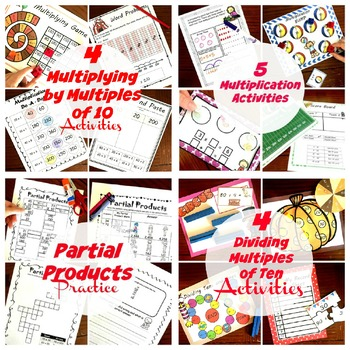# Multiplication and Division BundleResource Type
File Type
Zip (48 MB|100 +)
Standards
\$20.50
Bundle
List Price:
\$34.50
You Save:
\$14.00
Products in this Bundle (10)

showing 1-5 of 10 products

• Bundle Description
• Standards

This is a growing bundle, and more will be added to it in the coming months.

Right now, there is.....

1. Skip counting bundle that has children practicing skip counting up to 15 in five different ways. (Games, puzzles, clip cards, etc)

2. Next, children are introduced to multiplication with three ways to work on their facts, and two ways to explore and model multiplication.

3. There is a division bundle to work on basic division facts.

4. Next, the children practice multiplying by multiples of tens.

5. There are five activities for children to practice dividing by 10.

6. Then there are interactive notebooks for helping children learn how to multiple with partial products, lattice, arrrays, etc.

7. There is a short bundle for children to practice using partial products to solve problems.

8. Seven activities to practice Multiplication by two and three digit practice

8. And there is a division bundle for working on dividing multiples of 10's by ones.

What is coming????

1. Multiplication by two and three digit practice

2. Long division practice

Multiply or divide to solve word problems involving multiplicative comparison, e.g., by using drawings and equations with a symbol for the unknown number to represent the problem, distinguishing multiplicative comparison from additive comparison.
Interpret a multiplication equation as a comparison, e.g., interpret 35 = 5 × 7 as a statement that 35 is 5 times as many as 7 and 7 times as many as 5. Represent verbal statements of multiplicative comparisons as multiplication equations.
Fluently multiply and divide within 100, using strategies such as the relationship between multiplication and division (e.g., knowing that 8 × 5 = 40, one knows 40 ÷ 5 = 8) or properties of operations. By the end of Grade 3, know from memory all products of two one-digit numbers.
Understand division as an unknown-factor problem. For example, find 32 ÷ 8 by finding the number that makes 32 when multiplied by 8.
Determine the unknown whole number in a multiplication or division equation relating three whole numbers. For example, determine the unknown number that makes the equation true in each of the equations 8 × ? = 48, 5 = __ ÷ 3, 6 × 6 = ?.
Total Pages
100 +